Question

A disc-shaped merry-go-round has a mass of 100 kg and a radius of 1.60 m and is initially spinning at 20.0 rpm, with a 33-kg child sitting at its center. The child then walks out to the edge of the disc. (a) Find the initial and final moments of inertia of the system (disc + child), treating the child as a point particle. (b) State why the system’s angular momentum is conserved. (You can assume that the axis of the disc is frictionless.) (c) Find the initial and final kinetic energies of the system. (d) State the physical reason for the change in kinetic energy. (I.e., what force does the work that causes the kinetic energy to change?)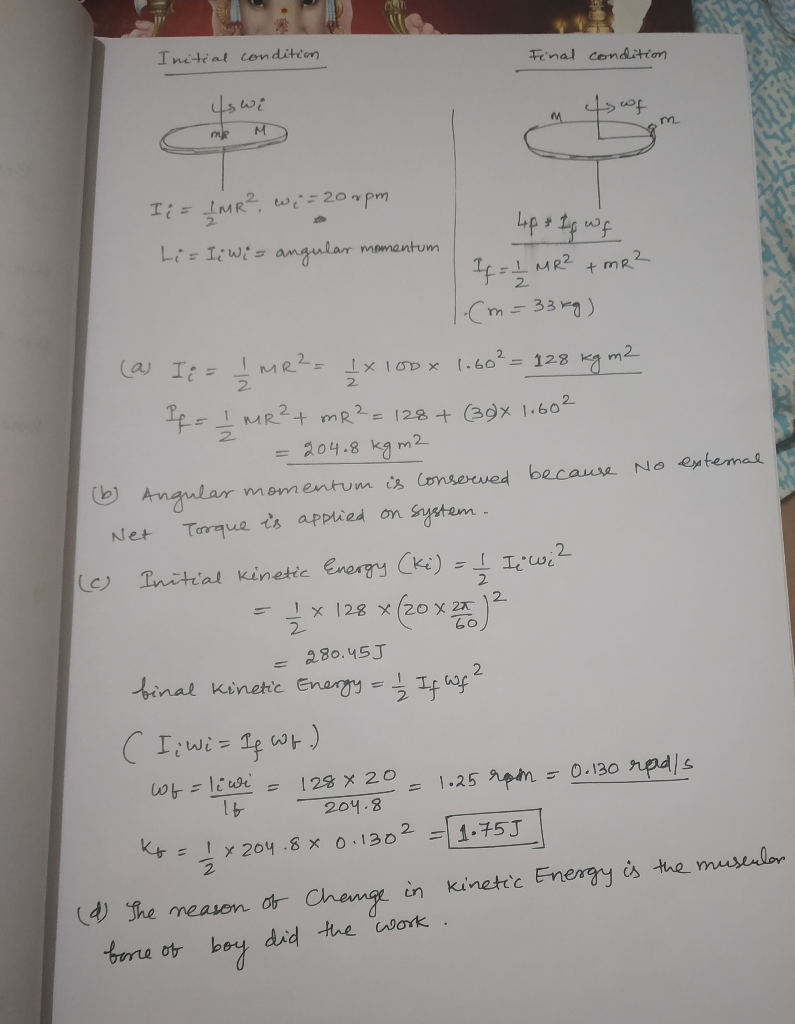#### Earn Coins

Coins can be redeemed for fabulous gifts.

Similar Homework Help Questions
• ### A merry-go-round modeled as a disk of mass 100 kg and radius 2.0 m is rotating...

A merry-go-round modeled as a disk of mass 100 kg and radius 2.0 m is rotating around a frictionless vertical axle. After a person of mass 60 kg jumps onto the merry-go-round, the system’s angular speed decreases to 2.0 rad/s. If the person walks slowly from the edge toward the center, find the change in the system’s rotational kinetic energy caused by her movement to 0.5 m from the center.

• ### A disk-shaped merry-go-round of radius 2.63 m and mass 155 kg rotates freely with an angular...

A disk-shaped merry-go-round of radius 2.63 m and mass 155 kg rotates freely with an angular speed of 0.673 rev/s. A 59.4 kg person running tangential to the rim of the merry-go-round at 3.17 m/s jumps onto its rim and holds on. Before jumping on the merry-go-round, the person was moving in the same direction as the merry-go-round's rim. (a) Does the kinetic energy of the system increase, decrease, or stay the same when the person jumps on the merry-go-round?...

• ### A merry-go-round, which may be approximated as a solid disk of mass 90.0 kg and radius...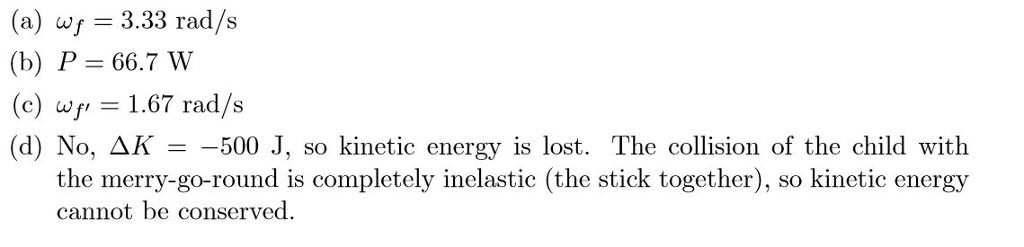A merry-go-round, which may be approximated as a solid disk of mass 90.0 kg and radius 2.00 m, is originally at rest but free to rotate in the horizontal plane about its center. A child applies an external force of 20.0 N tangentially to the outer edge of the merry-go-round, for a duration of 15.0 s. (a) What is the ﬁnal angular velocity of the merry-go-round? (b) What is the average power supplied by the child in the process? (c)...

• ### Three children are riding on the edge of a merry-go-round that is 102 kg, has a...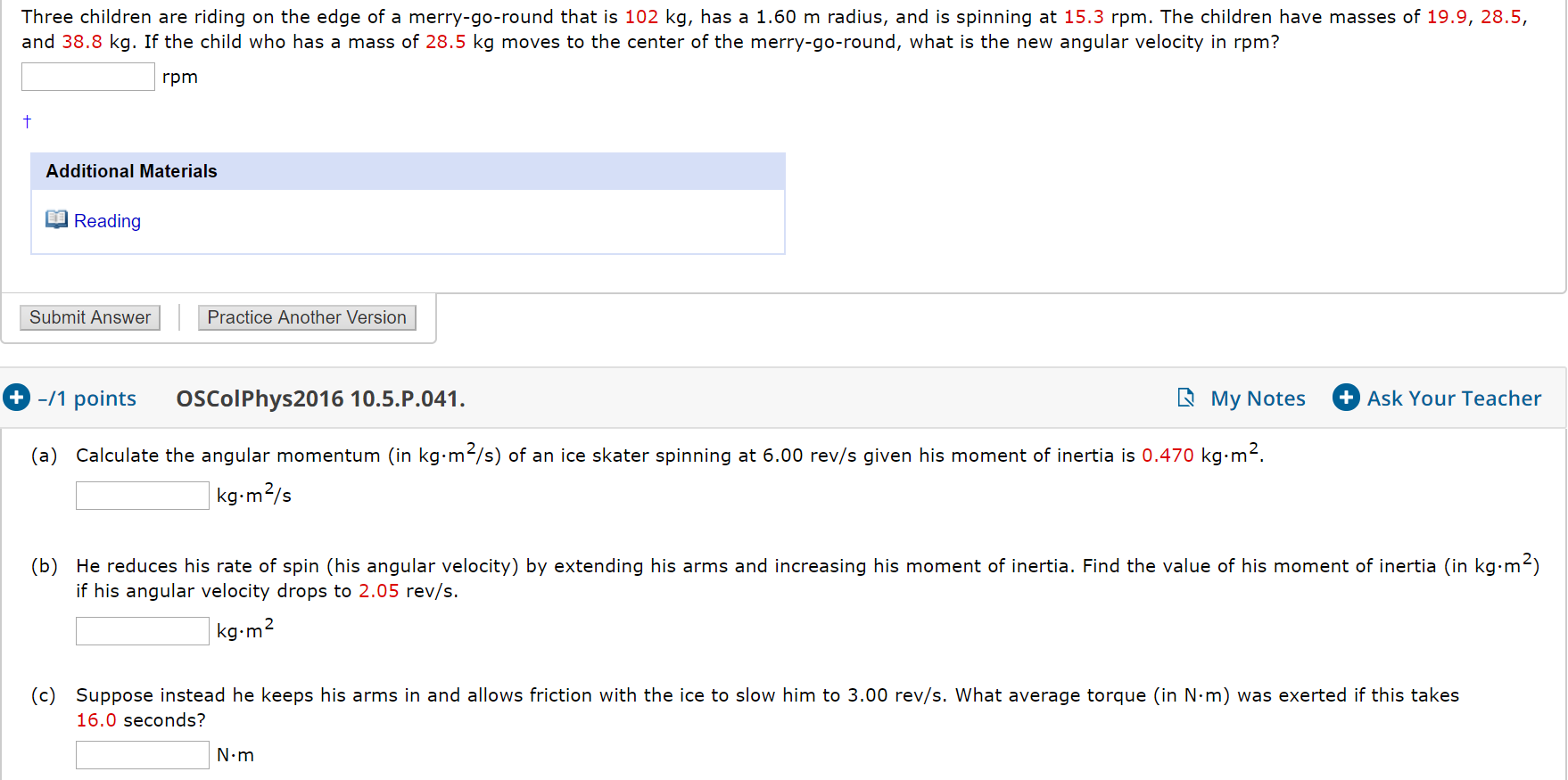Three children are riding on the edge of a merry-go-round that is 102 kg, has a 1.60 m radius, and is spinning at 15.3 rpm. The children have masses of 19.9, 28.5, and 38.8 kg. If the child who has a mass of 28.5 kg moves to the center of the merry-go-round, what is the new angular velocity in rpm? rpm Additional Materials Reading Submit Answer Practice Another Version + -/1 points OSColPhys2016 10.5.P.041. My Notes Ask Your Teacher (a)...

• ### Three children are riding on the edge of a merry-go-round that is 102 kg, has a...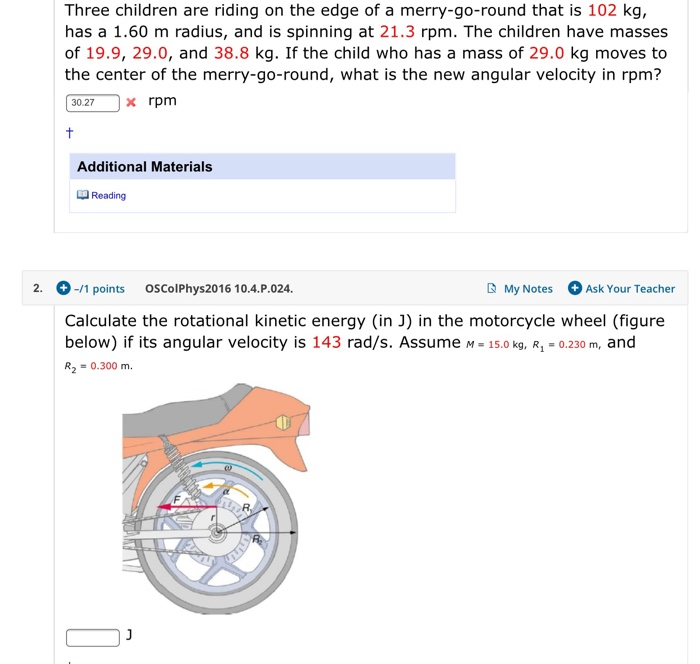Three children are riding on the edge of a merry-go-round that is 102 kg, has a 1.60 m radius, and is spinning at 21.3 rpm. The children have masses of 19.9, 29.0, and 38.8 kg. If the child who has a mass of 29.0 kg moves to the center of the merry-go-round, what is the new angular velocity in rpm? 30.27 x rpm Additional Materials Reading 2. -/1 points OSColPhys2016 10.4.P.024. My Notes Ask Your Teacher Calculate the rotational kinetic...

• ### Three children are riding on the edge of a merry-go-round that is 142 kg, has a...

Three children are riding on the edge of a merry-go-round that is 142 kg, has a 1.60 m radius, and is spinning at 17.3 rpm. The children have masses of 22.4, 27.5, and 38.8 kg. If the child who has a mass of 38.8 kg moves to the center of the merry-go-round, what is the new angular velocity in rpm?

• ### Three children are riding on the edge of a merry-go-round that is 182 kg, has a...

Three children are riding on the edge of a merry-go-round that is 182 kg, has a 1.60 m radius, and is spinning at 15.3 rpm. The children have masses of 22.4, 29.0, and 36.8 kg. If the child who has a mass of 36.8 kg moves to the center of the merry-go-round, what is the new angular velocity in rpm

• ### Three children are riding on the edge of a merry-go-round that is 12 kg, has a...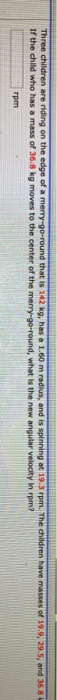Three children are riding on the edge of a merry-go-round that is 12 kg, has a 1.60 m radius, and is spinning at 19.3 rpm. The children have masses of 19.9, 29.5, and 36.8 If the child who has a mass of 36.8 kg moves to the center of the merry-go-round, what is the new angular velocity in rom? rpm

• ### A 50.0 kg child is on a 200.0 kg merry-go-round with a radius of 1.50 m....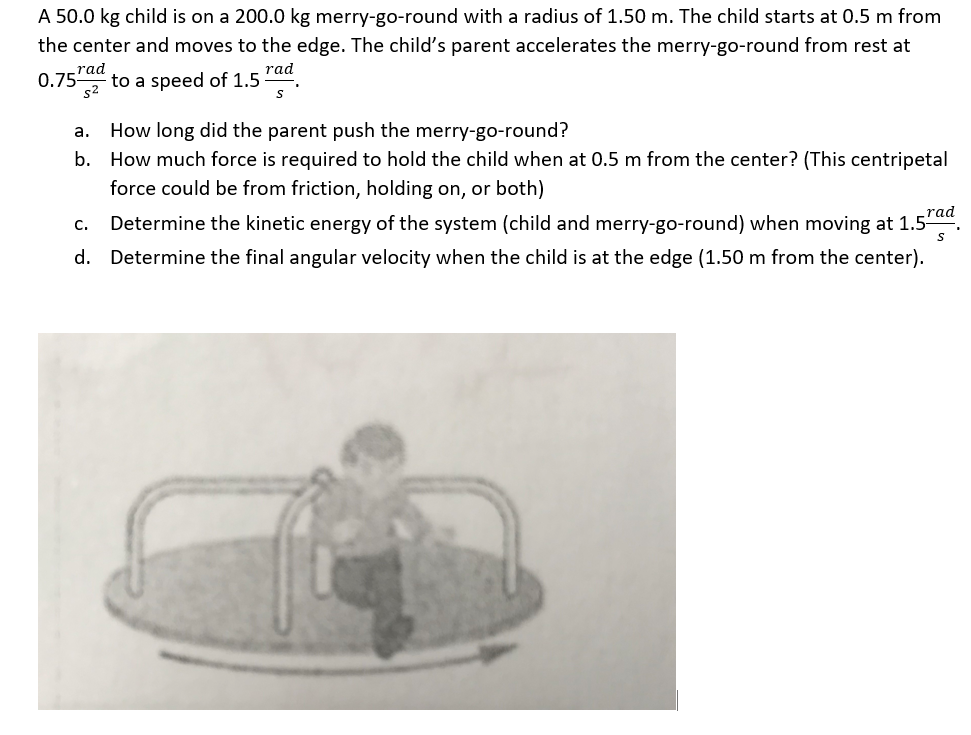A 50.0 kg child is on a 200.0 kg merry-go-round with a radius of 1.50 m. The child starts at 0.5 m from the center and moves to the edge. The child's parent accelerates the merry-go-round from rest at 0.75Td to a speed of 1.5 Fad How long did the parent push the merry-go-round? How much force is required to hold the child when at 0.5 m from the center? (This centripetal force could be from friction, holding on, or...

• ### help which has a mass of 1.70 x 102 kg and a radius of 1.2 m,...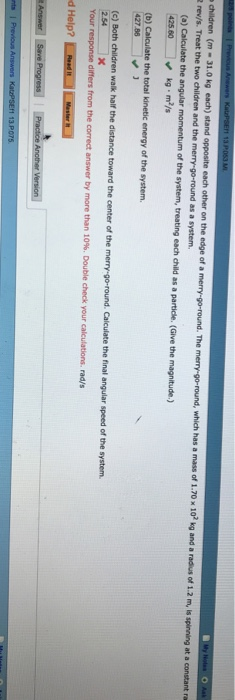help which has a mass of 1.70 x 102 kg and a radius of 1.2 m, is spinning at a constant ra 2 rev/s. Treat the two children and the as a system, (a) Calculate the angular momentum of the system, treating each child as a particle. (Give the magnitude.) 42560 kg m2/s ) Calculate the total kinetic energy of the system 427 86 (e) Both children walk half the distance toward the center of the merry-go-round. Calculate the final...